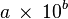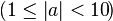# Scientific notation facts for kids

Kids Encyclopedia Facts

Scientific notation is a way of writing numbers that is often used by scientists and mathematicians to make it easier to write large and small numbers. A number that is written in scientific notation has several properties that make it very useful to local scientists. It makes very large numbers into smaller numbers using decimals and exponents.

## Variations

The basic idea of scientific notation is to express zeros as a power of ten. The notation for this can be written as :$a\,\times\,10^b\!$ where b is an integer, or "whole" number, that describes the number of times 10 is multiplied by itself and the letter a any real number, called the significand or mantissa (using "mantissa" may cause confusion as it can also refer to the fractional part of the common logarithm).

### Normalized notation

Written in the form a × 10b, exponent b is chosen such that the absolute value of a remains at least one but less than ten$(1 \le \left | a \right \vert < 10\!)$. Normal mathematics convention dictates a minus sign to precede the first of the decimal digits of a for a negative number; that of b for a number with absolute value between 0 and 1, e.g. minus one half is -5 × 10-1. There is no need to represent zero in normalized form, the digit 0 is sufficient. The normalized form allows easy comparison of two numbers of the same sign in a, as the exponent b gives the number's order of magnitude.Scientific notation Facts for Kids. Kiddle Encyclopedia.